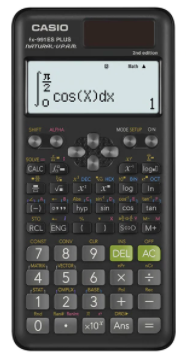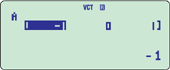# Casio FX-991ES Plus II Non Programmable Scientific Calculator #C79*Image may differ with actual product's layout, color, size & dimension. No claim will be accepted for image mismatch.

# Casio FX-991ES Plus II Non Programmable Scientific Calculator #C79

Product Id: 130.01.685.50

Regular Price  1,600
Special Price  1,480

Delivery time?

Quick Overview

Model - Casio FX-991ES Plus II
Brand - Casio
Type - Non Programmable Scientific Calculator
Display - Dot matrix display
Digit - 12 digits (10 + 2 digits)
Key - Plastic keys
Model
Casio FX-991ES Plus II
Type
Non Programmable Scientific Calculator
Display
Dot matrix display
Digit
12 digits (10 + 2 digits)
Key
Plastic keys
Number of Functions
417
Power Sources
Solar & Battery
Dimensions
161.5 x 77 x 11.1mm
Weight (Kg)
95gm
Specialty
Non Programmable Scientific Calculator, Natural textbook display, Display expression same as textbook, Solar & Battery, Solar powered when light is sufficient, battery powered when light is insufficient, Simple and easy to use, Optimal education tools realized through s design that emphasizes comfort in the hand, legibility, and usability through the pursuit of simplicity, Features Natural Textbook Display format for displaying equations and calculation result just as they appear in textbooks, Authentic quality, Excellent quality and authentication ensure confidence and peace of mind for use in educational settings, Random integers, Fraction calculations, Combination and permutation, Statistics (List-based STAT data editor, standard deviation, regression analysis), 9 variables, Table function, Comes with new slide-on hard case, Equation calculations, Integration/differential calculations, Matrix calculations, Vector calculations, Complex number calculations, CALC function, SOLVE function, Complex number calculations, Differential, Integration, 10 + 2 digits, Dot matrix display, Multi-replay, Plastic keys, Basic Mathematical Functions, Base-n calculation, Scientific Constants, Metric conversion function, List based STAT-data editor, Non Graphing, Differentiation, Matrix, Equation
Others
Part No: C79, Natural textbook display: Display expression same as textbook, 10 + 2 digits: 10-digit mantissa + 2-digit exponential display, Dot matrix display: High-resolution screen provides beautiful looking graphs every time, Multi-replay: Quick and easy recall of previously executed formulas for editing and re-execution, Solar & Battery: Solar powered when light is sufficient, battery powered when light is insufficient, Plastic keys: Designed and engineered for easy operation, Basic Mathematical Functions: Trigonometric, Exponential logarithmic, etc., Table Generation: can create a numerical table based on that expression by registering a function expression. In addition, graph functions can be represented by graphs, Base-n calculation: Binary/Octal/Hexadecimal, Scientific Constants: can use constant symbols used in physics, science classes, Metric conversion function: can convert a number in one unit to a number in a different unit, Basic Statistics: Standard statistics functions such as Mean, SUM, Standard Deviation, and Regression, List based STAT-data editor: Viewing and editing of input data in list format, showing data groups (x-data, y-data, frequency) and surrounding data
Warranty
3 year
Made in/ Assemble
Thailand
Country Of Origin
Japan

## fx-991ES PLUS-2### Simple and easy to use

Optimal education tools realized through s design that emphasizes comfort in the hand, legibility, and usability through the pursuit of simplicity

### Natural Textbook Display

Features Natural Textbook Display format for displaying equations and calculation result just as they appear in textbooks

### Authentic quality

Excellent quality and authentication ensure confidence and peace of mind for use in educational settings

### Main functions

• Random integers
• Fraction calculations
• Combination and permutation
• Statistics (List-based STAT data editor, standard deviation, regression analysis)
• 9 variables
• Table function
• Comes with new slide-on hard case
• Equation calculations
• Integration/differential calculations
• Matrix calculations
• Vector calculations
• Complex number calculations
• CALC function
• SOLVE function
• Base-n calculation

IntegrationComplex number calculationsDifferentialVectorMatrix operationsModel - Casio FX-991ES Plus II, Brand - Casio, Type - Non Programmable Scientific Calculator, Display - Dot matrix display, Digit - 12 digits (10 + 2 digits), Number of Functions - 417, Key - Plastic keys, Power source - Solar & Battery, Dimension - 161.5 x 77 x 11.1mm, Weight - 95gm, Others - Part No: C79, Natural textbook display: Display expression same as textbook, 10 + 2 digits: 10-digit mantissa + 2-digit exponential display, Dot matrix display: High-resolution screen provides beautiful looking graphs every time, Multi-replay: Quick and easy recall of previously executed formulas for editing and re-execution, Solar & Battery: Solar powered when light is sufficient, battery powered when light is insufficient, Plastic keys: Designed and engineered for easy operation, Basic Mathematical Functions: Trigonometric, Exponential logarithmic, etc., Table Generation: can create a numerical table based on that expression by registering a function expression. In addition, graph functions can be represented by graphs, Base-n calculation: Binary/Octal/Hexadecimal, Scientific Constants: can use constant symbols used in physics, science classes, Metric conversion function: can convert a number in one unit to a number in a different unit, Basic Statistics: Standard statistics functions such as Mean, SUM, Standard Deviation, and Regression, List based STAT-data editor: Viewing and editing of input data in list format, showing data groups (x-data, y-data, frequency) and surrounding data, Specialty - Non Programmable Scientific Calculator, Natural textbook display, Display expression same as textbook, Solar & Battery, Solar powered when light is sufficient, battery powered when light is insufficient, Simple and easy to use, Optimal education tools realized through s design that emphasizes comfort in the hand, legibility, and usability through the pursuit of simplicity, Features Natural Textbook Display format for displaying equations and calculation result just as they appear in textbooks, Authentic quality, Excellent quality and authentication ensure confidence and peace of mind for use in educational settings, Random integers, Fraction calculations, Combination and permutation, Statistics (List-based STAT data editor, standard deviation, regression analysis), 9 variables, Table function, Comes with new slide-on hard case, Equation calculations, Integration/differential calculations, Matrix calculations, Vector calculations, Complex number calculations, CALC function, SOLVE function, Complex number calculations, Differential, Integration, 10 + 2 digits, Dot matrix display, Multi-replay, Plastic keys, Basic Mathematical Functions, Base-n calculation, Scientific Constants, Metric conversion function, List based STAT-data editor, Non Graphing, Differentiation, Matrix, Equation, Warranty - 3 year, Country of Origin - Japan, Made in/ Assemble - Thailand

## Your review

by Gigi Hadid

very good## Your Question